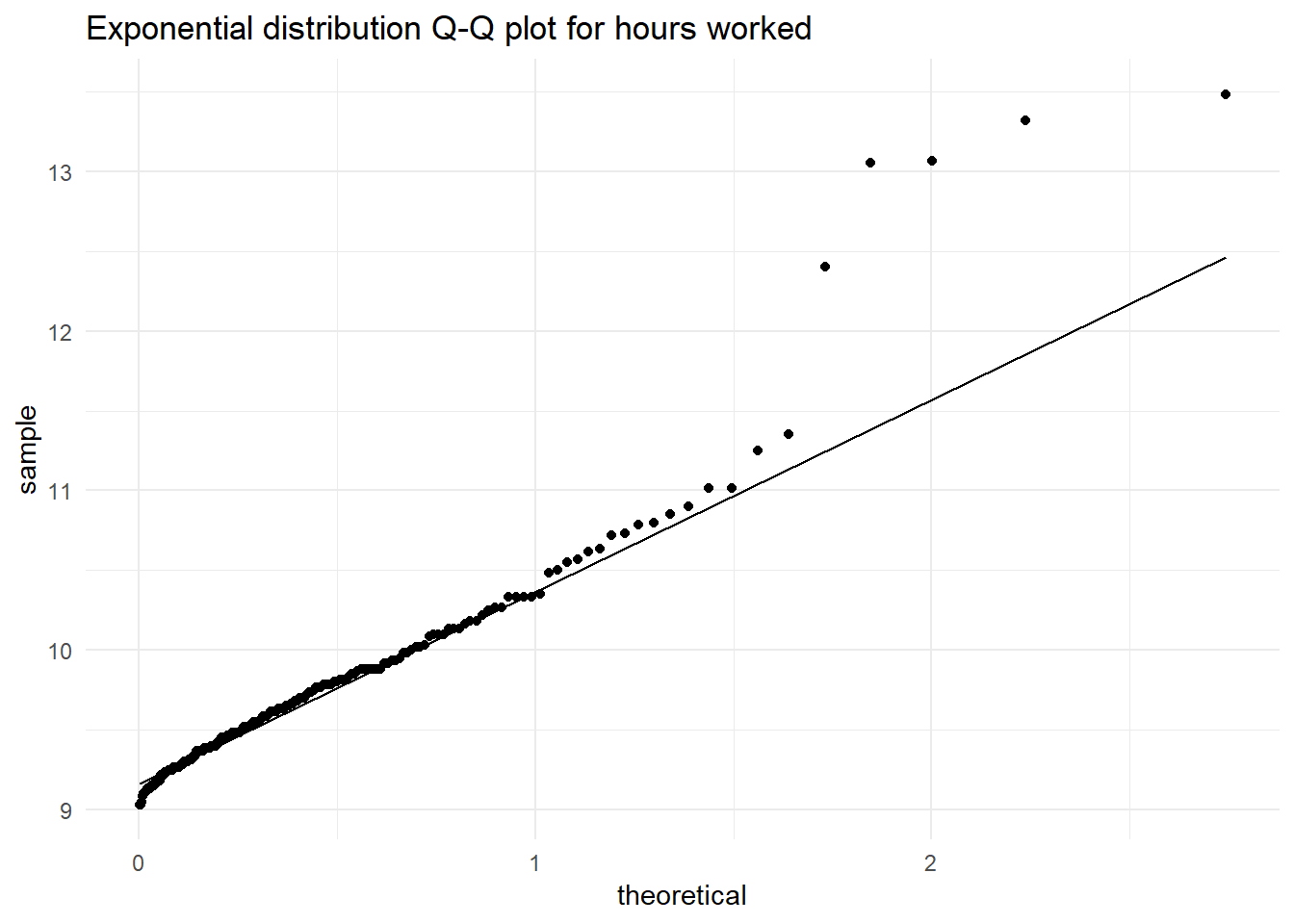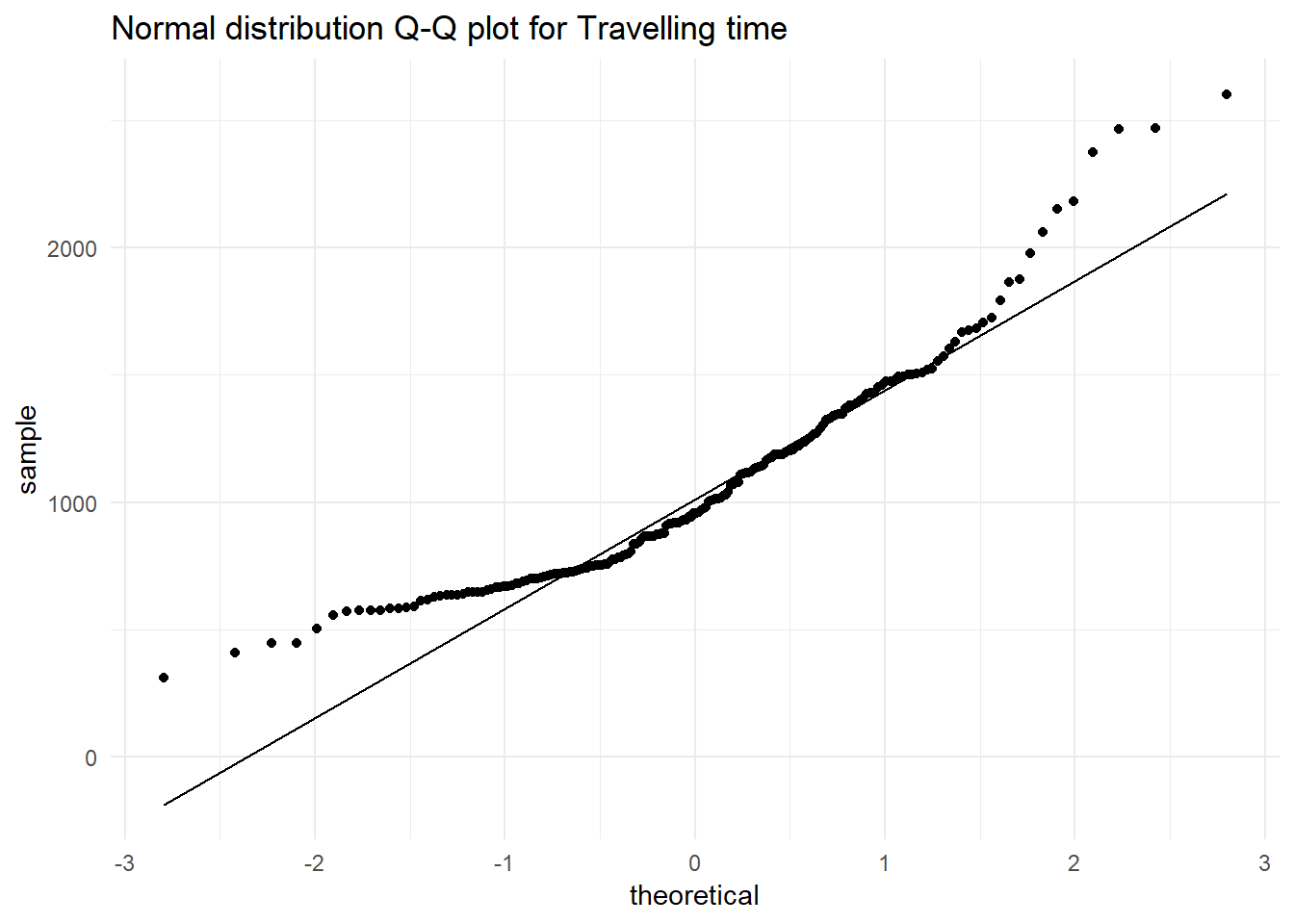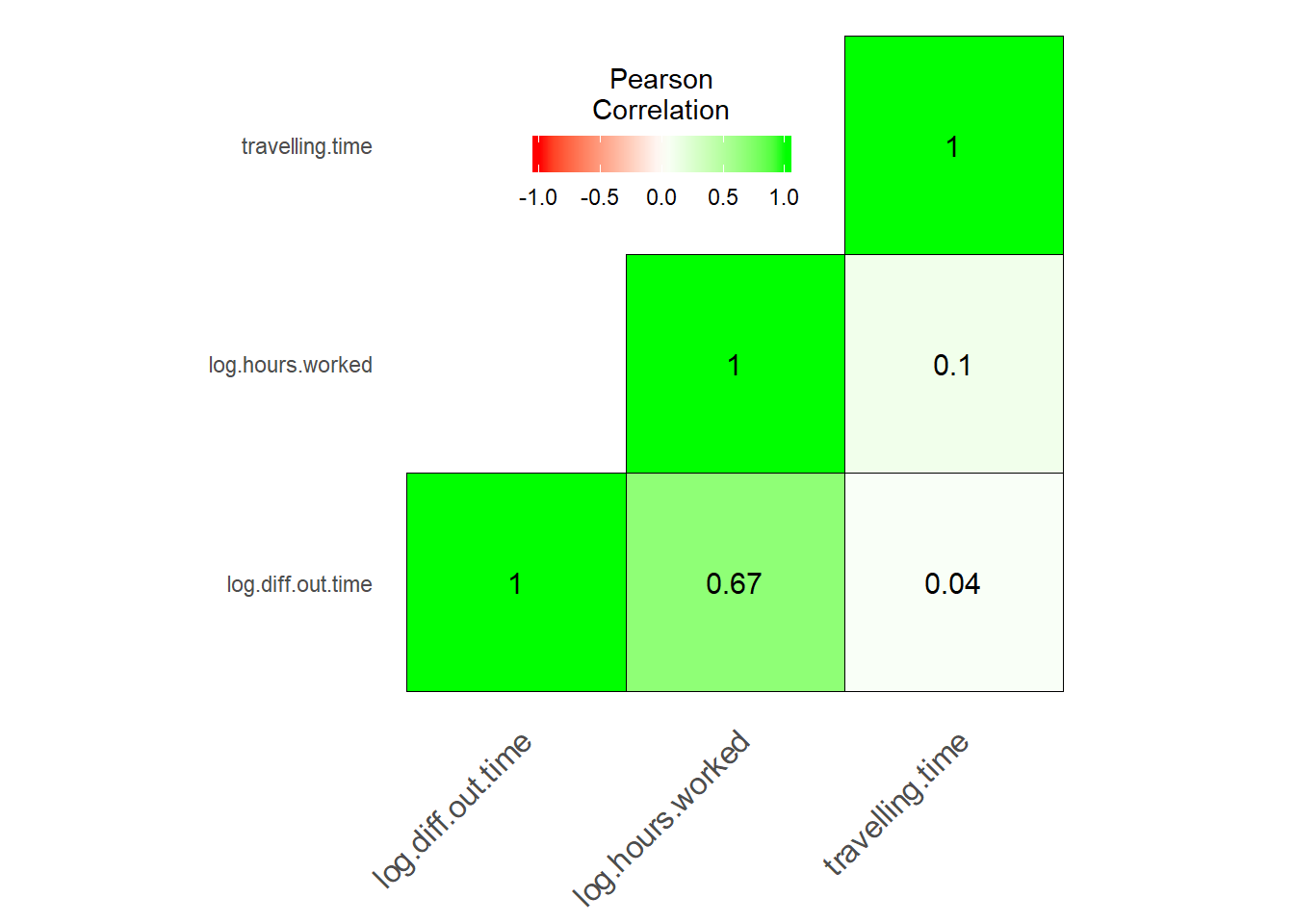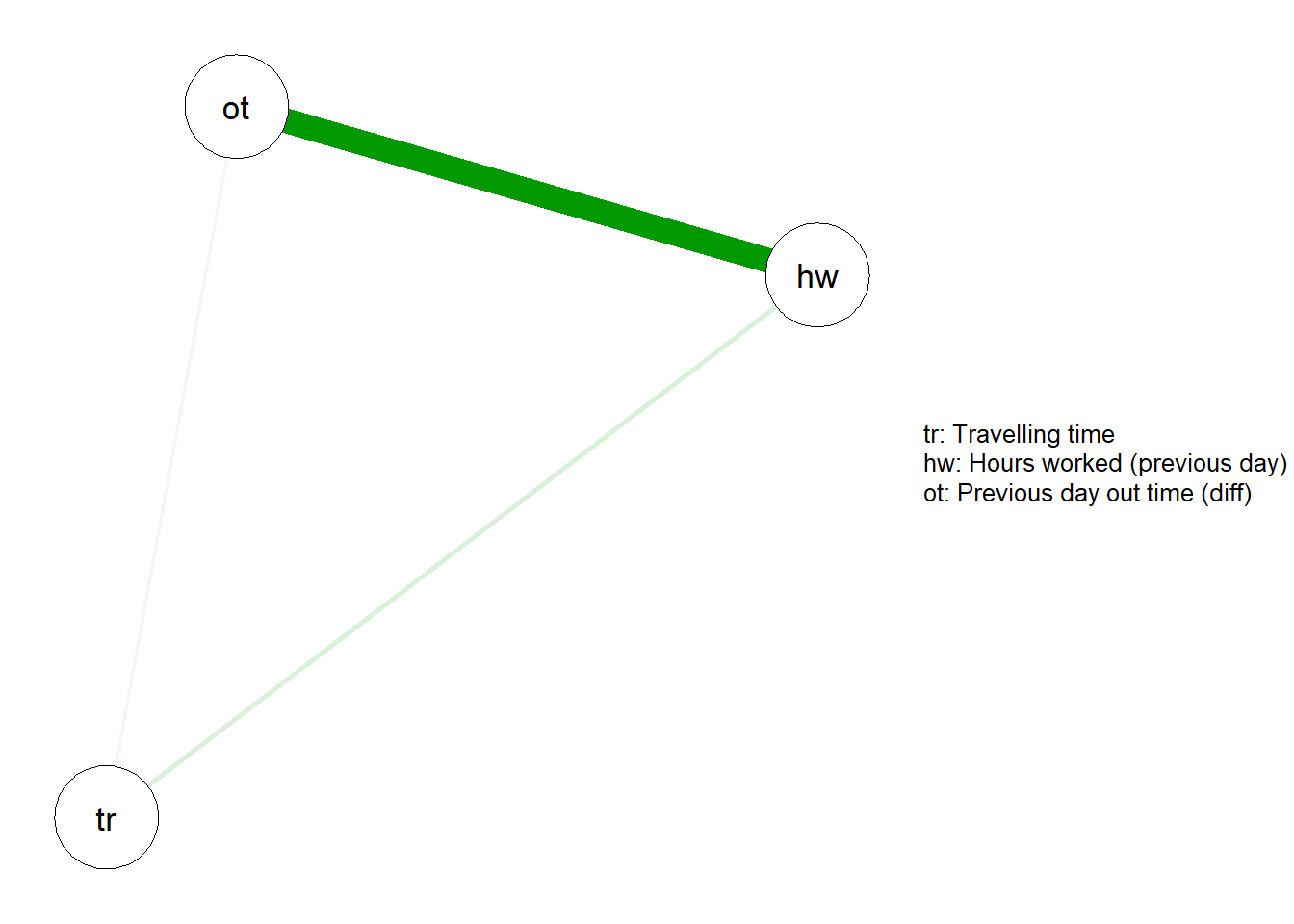I want to go thru some basic statistical concepts before continuing with the EDA and in-time problem. I want to explain what is multicollinearity and how and when to tackle it.

## Collinearity

Collinearity is a linear association between two variables. Two variables are collinear if there is a linear relationship between them. For example, $$X_{1}$$ and $$X_{2}$$ are perfectly collinear if there exist parameters $$\lambda _{0}$$ and $$\lambda _{1}$$ such that, for all observations i, I have

$X_{2i} = \lambda_0 + \lambda_1 X_{1i}$ Here $$\lambda _{1}$$ can be considered as the slope of the equation and $$\lambda _{0}$$ is the intercept.

### Correlation

Correlation is a numerical measure of how close two variables are having a linear relationship with each other. The most popular form of the correlation coefficient is Pearson’s coefficient($$r_{xy}$$).

#### Pearson’s product-moment coefficient

Pearson’s product-moment coefficient is the most common correlation coefficient. It is obtained by dividing the covariance of the two variables by the product of their standard deviations.

$r_{xy}=\mathrm {corr} (X,Y)={\mathrm {cov} (X,Y) \over \sigma _{X}\sigma _{Y}} = {\frac {\sum \limits _{i=1}^{n}(x_{i}-{\bar {x}})(y_{i}-{\bar {y}})}{(n-1)s_{x}s_{y}}}={\frac {\sum \limits _{i=1}^{n}(x_{i}-{\bar {x}})(y_{i}-{\bar {y}})}{\sqrt {\sum \limits _{i=1}^{n}(x_{i}-{\bar {x}})^{2}\sum \limits _{i=1}^{n}(y_{i}-{\bar {y}})^{2}}}},$ where x and y are the means of X and Y, and $$s_{x}, s_{y}$$ are the standard deviations of X and Y.

The Pearson correlation is $$+1$$ in case of a perfect direct(increasing) linear relationship (correlation), $$-1$$ in the case of a perfect decreasing (inverse) linear relationship(anti-correlation), and some value in the open interval $$(-1, +1)$$ in all other cases, indicating the degree of linear dependence between the variables. As it approaches zero there is less of a relationship(closer to uncorrelated). The closer the coefficient is to either $$-1$$ or $$+1$$, the stronger is the correlation between the variables.

If the variables are independent, Pearson’s correlation coefficient is 0, but the converse is not true because the correlation coefficient detects only linear dependencies between two variables. For example, suppose the random variable X is symmetrically distributed about zero, and $$Y = X^{2}$$. Then Y is completely determined by X, so that X and Y are perfectly dependent, but their correlation is zero.

## Multicollinearity

Multicollinearity occurs when independent variables in a regression model are correlated. This correlation is a problem because independent variables should be independent. If the degree of correlation between variables is high, it can cause problems when I fit the model and interpret the results.

A key goal of regression (or classification) analysis is to isolate the relationship between each independent variable and the dependent variable. The interpretation of a regression coefficient is that it represents the mean change in the dependent variable for each 1 unit change in an independent variable when I hold all of the other independent variables constant. That last portion is crucial for our discussion about multicollinearity.

The idea is that I can change the value of one independent variable and not the others. However, when independent variables are correlated, it indicates that changes in one variable are associated with shifts in another variable. The stronger the correlation, the more difficult it is to change one variable without changing another. It becomes difficult for the model to estimate the relationship between each independent variable and the dependent variable independently because the independent variables tend to change in unison.

### What problems do multicollinearity cause?

1. The coefficients become very sensitive to small changes in the model.
2. I will not be able to trust the p-values to identify independent variables that are statistically significant.

That said, I need not always fix multicollinearity. Multicollinearity affects the coefficients and p-values, but it does not influence the predictions, precision of the predictions, and the goodness-of-fit statistics. If my primary goal is to make predictions, and I don’t need to understand the role of each independent variable, I don’t need to reduce severe multicollinearity.

# In-time analysis problem

Now that I have discussed this much, let me continue with the in-time analysis problem. I want to analyze my entry time at office and understand how different factors affect it.

Collinearity checks are done after Univariate and multivariate analysis in EDA. One reason for doing so is that now I know how each factor looks like, and as collinearity is only a linear association between two explanatory variables, I can convert my factors to linear after applying some functions on them(log, square root etc).

After multivariate analysis, I currently have the factors
1. date (month / weekday / season etc)
2. main_activity (means of transport)
3. hours.worked (of the previous day)
4. travelling.time (time it took to travel from house to office)
5. home.addr (the place of residence)
6. diff.out.time (previous day out of office time)

Out of these factors, only travelling.time, hours.worked and diff.out.time are continuous variables, so, for now, I will restrict my analysis to these three factors.

The dependent variable is diff.in.time(the difference between my actual in time vs policy in-time)

Sample Data
diff.in.time date main_activity hours.worked travelling.time home.addr diff.out.time
75 34 2018-04-06 IN_VEHICLE 9.450000 774.642 Old House -7
26 22 2018-01-10 ON_FOOT 9.500000 308.578 Old House 8
88 17 2018-04-25 ON_FOOT 10.350000 1015.017 Old House 64
179 -7 2018-10-30 ON_BICYCLE 10.566667 1668.824 New House 101
123 -12 2018-07-11 IN_VEHICLE 9.533333 866.401 Old House 44

As a recap on univariate analysis, hours.worked and diff.in.out.time were exponential distributions(while checking for collinearity I will take their log for linear relationships),while travelling.time is close to a normal distribution(with exponential tails, but I will ignore that for now)## Testing for collinearity

One of the most popular tests to check multicollinearity is VIF factor(which will be discussed and calculated in a different blog post). What I want to instead do is visually see how the independent factors influence one another. I can do it in two main ways:

### Correlation matrix

A correlation matrix is a table showing correlation coefficients($$r_{xy}$$) between variables. Each cell in the table shows the correlation between the two variables.

First I will make a reusable correlation matrix plotting function.

corr_matrix_plotting_fxn <- function(df){
library(reshape)
library(ggplot2)

# Making a correlation matrix
cormat <- round(cor(df), 2)

# Getting the upper triangular matrix
cormat[upper.tri(cormat)]<- NA

# Melt the correlation data and drop the rows with NA values
melted_cormat <- reshape::melt(cormat, na.rm = TRUE)
colnames(melted_cormat) <- c('Var1', 'Var2', 'value')
melted_cormat <- melted_cormat %>% filter(!is.na(value))

# Plot the corelation matrix
ggheatmap <- ggplot(melted_cormat, aes(Var2, Var1, fill = value))+
geom_tile(color = "black")+
scale_fill_gradient2(low = "red", high = "green", mid = "white",
midpoint = 0, limit = c(-1,1), space = "Lab",
name="Pearson\nCorrelation") +
theme_minimal()+ # minimal theme
theme(axis.text.x = element_text(angle = 45, vjust = 1, size = 12, hjust = 1))+
coord_fixed() +
geom_text(aes(Var2, Var1, label = value), color = "black", size = 4) +
theme(
axis.title.x = element_blank(),
axis.title.y = element_blank(),
panel.grid.major = element_blank(),
panel.border = element_blank(),
panel.background = element_blank(),
axis.ticks = element_blank(),
legend.justification = c(1, 0),
legend.position = c(0.6, 0.7),
legend.direction = "horizontal")+
guides(fill = guide_colorbar(barwidth = 7, barheight = 1,
title.position = "top", title.hjust = 0.5))
print(ggheatmap)

}

In the above function, I will just input the data frame with the necessary columns(modified dependent variables) and I will get the plot.

# Selecting necessary columns after converting to linear data sets
corr.variables <- travel %>%
filter(diff.out.time > -15) %>%
mutate(log.hours.worked = log(hours.worked),
log.diff.out.time = log(diff.out.time + 15)) %>%
dplyr::select(travelling.time, log.hours.worked, log.diff.out.time)

# Plotting the matrix
corr_matrix_plotting_fxn(corr.variables)I can see that Hours worked in the previous day and the out-time of the previous day are slightly correlated(Which conceptually seems likely).

### Correlation Network

Using a correlation matrix I can only check if two variables are correlated. If multiple variables are correlated, I should use the Correlation Network. Even when only two variables are correlated, these plots tell me which variable among the two to keep and which to reject as a dependent variable in our model.

library("qgraph")

# Legend
Names <- c('Travelling time', 'Hours worked (previous day)', 'Previous day out time (diff)')

# Renaming variables (not necessary)
colnames(corr.variables) <- c('tr', 'hw', 'ot')

cormat <- round(cor(corr.variables), 2)
qgraph(cormat, graph = "pcor", layout = "spring", nodeNames = Names, legend.cex = 0.4)From this plot, I can see that traveling time is not correlated to any other variable, and among the other two which are correlated, I will pick out time difference as my second variable and reject hours worked.

#### Basic statistical formulas

The formulas for some basic statistical terms used in this blog are given below.

The equation for standard deviation. $\sigma = \sqrt{\frac{\sum\limits_{i=1}^{n} \left(x_{i} - \bar{x}\right)^{2}} {n-1}}$

The equation for covariance $cov_{x,y} = \frac{\sum\limits_{i=1}^{n}{(x_i-\overline{x}) \cdot (y_i-\overline{y})} }{n-1}$

Created using RMarkdown

|